# GenerationOfFMUs

Example to demonstrate variants to generate FMUs (Functional Mock-up Units)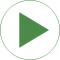# Information

This information is part of the Modelica Standard Library maintained by the Modelica Association.

This example demonstrates how to generate an input/output block (e.g. in form of an FMU - Functional Mock-up Unit) from various Rotational components. The goal is to export such an input/output block from Modelica and import it in another modeling environment. The essential issue is that before exporting it must be known in which way the component is utilized in the target environment. Depending on the target usage, different flange variables need to be in the interface with either input or output causality. Note, this example model can be used to test the FMU export/import of a Modelica tool. Just export the components marked in the icons as "toFMU" as FMUs and import them back. The models should then still work and give the same results as a pure Modelica model.

Connecting two inertias
The upper part (DirectInertia, InverseInertia) demonstrates how to export two inertias and connect them together in a target system. This requires that one of the inertias (here: DirectInertia) is defined to have states and the angle, angular velocity and angular acceleration are provided in the interface. The other mass (here: InverseInertia) is moved according to the provided input angle, angular velocity and angular acceleration.

Connecting a force element that needs angles and angular velocities
The middle part (SpringDamper) demonstrates how to export a force element that needs both angles and angular velocities for its force law and connect this force law in a target system between two inertias.

Connecting a force element that needs only angles
The lower part (Spring) demonstrates how to export a force element that needs only angles for its force law and connect this force law in a target system between two inertias.

# Components (15)

sine1 directInertia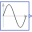Type: Sine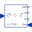Type: DirectInertia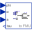Type: InverseInertia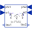Type: SpringDamper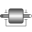Type: Inertia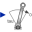Type: Torque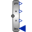Type: TorqueToAngleAdaptorType: InertiaType: TorqueToAngleAdaptor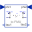Type: SpringType: InertiaType: TorqueType: TorqueToAngleAdaptorType: InertiaType: TorqueToAngleAdaptor